Rhombic dodecahedron made by a cube and six pyramids

We already know that the Rhombic Dodecahedron is related with honeycombs:We want to close a hexagonal prism as bees do, using three rhombi. Then, which is the shape of these three rhombi that closes the prism with the minimum surface area?.

And that you can build a Rhombic Dodecahedron addign six pyramids to a cube:Adding six pyramids to a cube you can build new polyhedra with twenty four triangular faces. For specific pyramids you get a Rhombic Dodecahedron that has twelve rhombic faces.

Cundy and Rollet wrote: "The rhombic dodecahedron and the cube. If a cube is divided by the six diametral planes which pass through pairs of opposite edges, it breaks up into six square pyramids. If these pyramids are assembled outwards on the faces of another cube, the result is a rhombic dodecahedron." (Cundy and Rollet, pag. 122)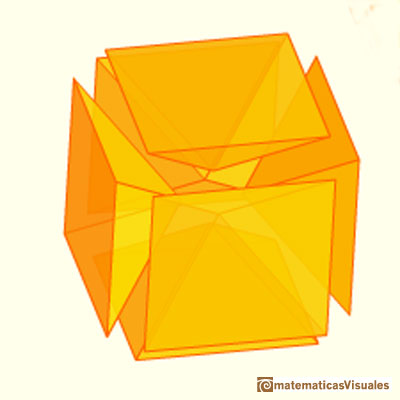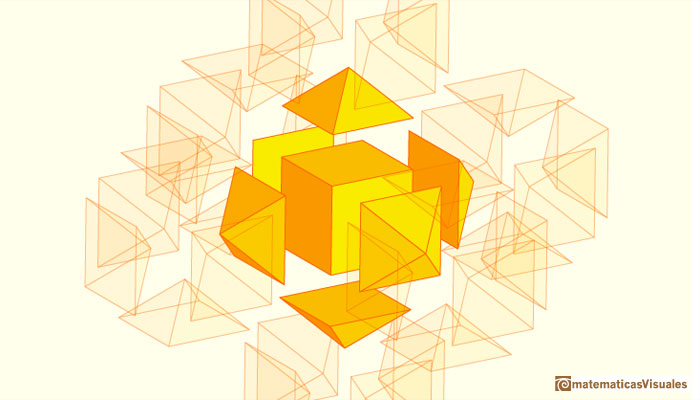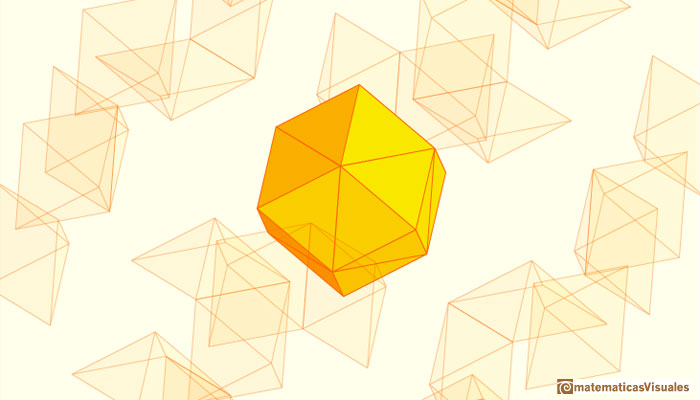The cube is dissected into six congruent square-based pyramids. Four edges of one pyramid are half the space diagonals of the cube.

This construction has several interesting consequences.

First, you can see in the interactive application that this six pyramids fill a cube. The volume of one of this pyramids is one-sixth of the volume of the cube.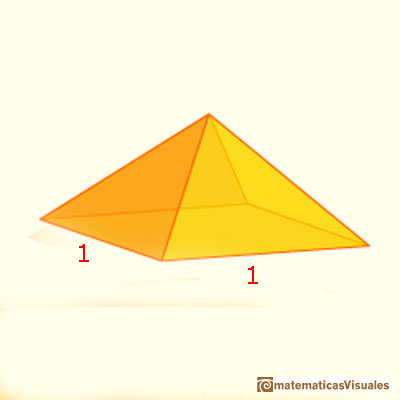Then you do not need the formula for the volume of a pyramid to calculate the volume of this particular pyramid.

By the way, the volume of a pyramid is one third of the base area times the perpendicular height. We can check that these pyramids verify the formula: their base area is 1 and the height is 1/2.

This is a very simple and important example of pyramid because after this example we can deduce the volume of pyramids with rectangular bases (expanding the cube in three directions).

The second consequence of this construction is that we can calculate the volume of a Rhombic Dodecahedron.

Remember that if inside the Rhombic Dodecahedron there is a unit cube, then the length of the edge of this Rhombic Dodecahedron is: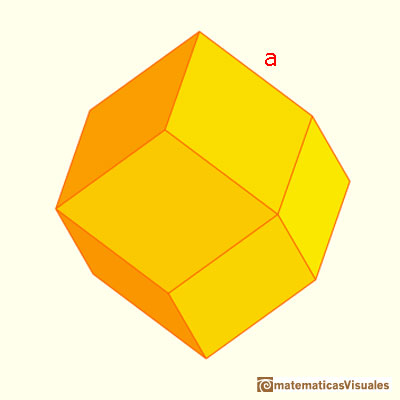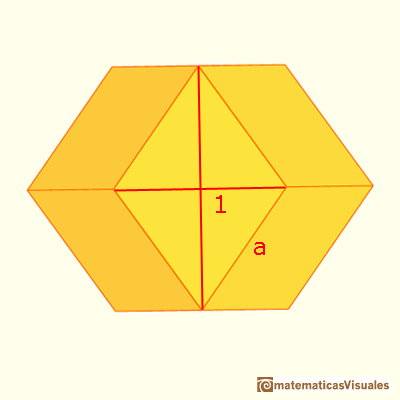Then the volume of this rhombic dodecahedron is twice the volume of the inside cube:

To calculate the volume if the edge length is 1:

Then,

The general formula for the volume of a rhombic dodecahedron of edge length a is:

You can play with the interactive application and see beautiful symmetries like these:The third consequence is that the Rhombic Dodecahedron fills the space.

We already know that we could build a beautiful box inspired in honeycombs.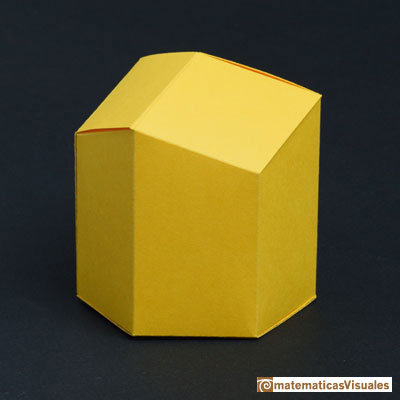Humankind has always been fascinated by how bees build their honeycombs. Kepler related honeycombs with a polyhedron called Rhombic Dodecahedron.

Now we can build a rhombic dodecahedron that is also a box.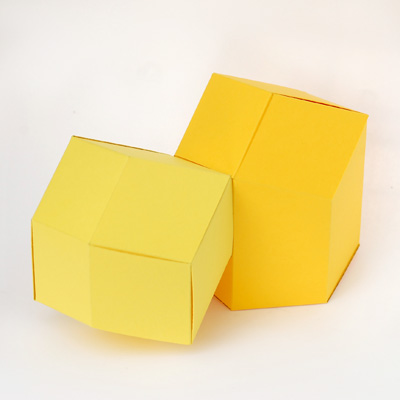You can download the template and build a model with cardboard. The designer of this box was John Edminster and you can find references in Beach Packaging Design.The rhombic dodecahedron is related with the cube. You can build a cube to put inside the rhombic dodecahedron. Inside the cube you can put a cuboctahedron (in this case, a omega star, a beautiful origami construction. Six pieces of paper are needed).

The rhombic dodecahedron is also related with the octahedron (you know that the cube and the octahedron are dual polyhedra). And you can build an octahedron and put it inside the dodecahedron.REFERENCES

Johannes Kepler - The Six Cornered Snowflake: a New Year's gif - Paul Dry Books, Philadelphia, Pennsylvania, 2010. English translation of Kepler's book 'De Nive Sexangula'. With notes by Owen Gingerich and Guillermo Bleichmar and illustrations by the spanish mathematician Capi Corrales Rodrigáñez.
D'Arcy Thompson - On Growth And Form - Cambridge University Press, 1942.
Hugo Steinhaus - Mathematical Snapshots - Oxford University Press - Third Edition.
Magnus Wenninger - 'Polyhedron Models', Cambridge University Press.
Peter R. Cromwell - 'Polyhedra', Cambridge University Press, 1999.
H.Martin Cundy and A.P. Rollet, 'Mathematical Models', Oxford University Press, Second Edition, 1961.
W.W. Rouse Ball and H.S.M. Coxeter - 'Matematical Recreations & Essays', The MacMillan Company, 1947.Humankind has always been fascinated by how bees build their honeycombs. Kepler related honeycombs with a polyhedron called Rhombic Dodecahedron.We want to close a hexagonal prism as bees do, using three rhombi. Then, which is the shape of these three rhombi that closes the prism with the minimum surface area?.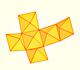A chain of six pyramids can be turned inwards to form a cube or turned outwards, placed over another cube to form the rhombic dodecahedron.The obtuse angle of a rhombic face of a Rhombic Dodecahedron is known as Maraldi angle. We need only basic trigonometry to calculate it.There are two essential different ways to pack spheres in an optimal disposition. One is related with the Rhombic Dodecaedron and the other to a polyhedron called Trapezo-rombic dodecahedron..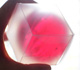Using a basic knowledge about the Rhombic Dodecahedron, it is easy to calculate the density of the optimal packing of spheres.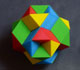Tetraxis is a wonderful puzzle designed by Jane and John Kostick. We study some properties of this puzzle and its relations with the rhombic dodecahedron. We can build this puzzle using cardboard and magnets or using a 3D printer.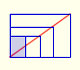There is a standarization of the size of the paper that is called DIN A. Successive paper sizes in the series A1, A2, A3, A4, and so forth, are defined by halving the preceding paper size along the larger dimension.Material for a session about polyhedra (Zaragoza, 9th May 2014). Simple techniques to build polyhedra like the tetrahedron, octahedron, the cuboctahedron and the rhombic dodecahedron. We can build a box that is a rhombic dodecahedron.Leonardo da Vinci made several drawings of polyhedra for Luca Pacioli's book 'De divina proportione'. Here we can see an adaptation of the truncated octahedron.Leonardo da Vinci made several drawings of polyhedra for Luca Pacioli's book 'De divina proportione'. Here we can see an adaptation of the cuboctahedron.A cuboctahedron is an Archimedean solid. It can be seen as made by cutting off the corners of a cube.A cuboctahedron is an Archimedean solid. It can be seen as made by cutting off the corners of an octahedron.The volume of an octahedron is four times the volume of a tetrahedron. It is easy to calculate and then we can get the volume of a tetrahedron.The truncated octahedron is an Archimedean solid. It has 8 regular hexagonal faces and 6 square faces. Its volume can be calculated knowing the volume of an octahedron.These polyhedra pack together to fill space, forming a 3 dimensional space tessellation or tilling.You can chamfer a cube and then you get a polyhedron similar (but not equal) to a truncated octahedron. You can get also a rhombic dodecahedron.A Cube can be inscribed in a Dodecahedron. A Dodecahedron can be seen as a cube with six 'roofs'. You can fold a dodecahedron into a cube.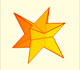If you fold the six roofs of a regular dodecahedron into a cube there is an empty space. This space can be filled with an irregular dodecahedron composed of identical irregular pentagons (a kind of pyritohedron).